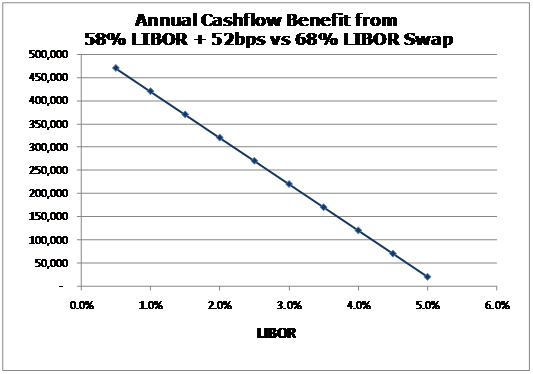# Are munis over-hedged with swaps? Pt3

Posted by Peter Orr on Oct 25, 2009

"It is better to understand a little, than to misunderstand a lot."

- Unknown

The prior two posts came to one simple conclusion: most tax-exempt issuers who have used LIBOR based swaps to hedge variable bonds are over-hedged (see prior posts for details why). This conclusion has two primary ramifications:

1. If you hedged with, for example, 15% more swap than necessary than the issuer paid 15% more to the swap dealer than necessary. Across an estimated \$1.5 billion+ in compensation to swap dealers over the last several years on these, its real dough
2. In high rate environments, the overall cost of funding will be lower than expected and in low rate environments higher

Let's look at a simple example. AnyCity, USA uses a 3.50% \$100 million 68% 1M LIBOR to hedge \$100 million in tax-exempt variable rate demand bonds (VRDBs). This 68% number was determined using an historic average and an implicit assumption of zero correlation between 1M LIBOR and SIFMA/1M LIBOR ratios. If one had assumed correlation of -.35, which is more consistent with what we've seen and might reasonably expect, then the right hedging index would be 58% LIBOR plus 0.52%. Both of these swaps carry a fixed rate of 3.5%.

When rates are low, the floating leg of the swap at 68% of 1M LIBOR is less than the 58% of LIBOR plus 52bps. On our \$100 million swap for AnyCity, the graph of LIBOR rate level versus annual benefit to having the 58%+52 basis point leg looks like this:Now obviously as rates go higher the benefit becomes a loss, but that's the point: this is no longer a hedged position as there's an inherent interest rate view within the structure. The overall synthetic fixed-rate structure (variable rate bonds swapped to fixed) performs worse than expected in low rate environments but better than expected in high rate environments. This is due to the fact that we expect SIFMA/LIBOR ratios to be somewhat higher on average in low rate environments and vice versa (the negative correlation between rates and ratios). What does this all mean? Well, few issuers are entering into new synthetic fixed rate deals so it matters more for those that are doing restructuring. What is the optimal portfolio-wide level of LIBOR based swaps for hedging tax-exempt variable rates? Probably less than one might think. What's an issuer to do? Well, if you have more swaps than you need than you could unwind some swaps now though in this rate environment they're likely under water. You could wait for rates to rise and unwind when the swaps are closer to a zero mark or even an asset. Have to be careful though...that'd be speculating.